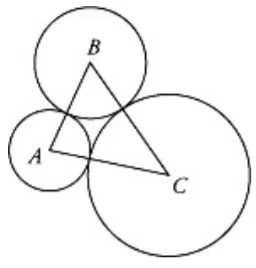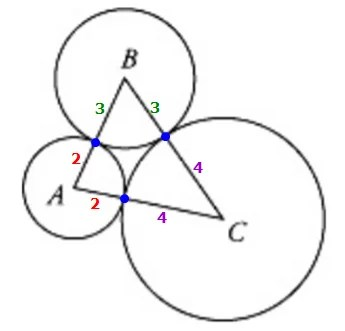Breaking News

# GMAT Problem Solving Questions with Answers – Test No. 3

GMAT Problem Solving Questions with Answers – Practice Test No. 1 for those who want to test their GMAT – Graduate Management Admission Test skills. GMAT is a standardized test used for the evaluation of students seeking admission in BBA, MBA, and post-graduate studies in Pakistan or abroad. Management studies provide you with the skills, preparation, and credentials you need to accelerate your career growth. MBA is the most popular degree program in Pakistan. LUMS and IBA MBA programs are the most applied in Pakistan.

Each quiz question in this course is made up of proven interesting researched concepts that test your awareness and grasp of the subject. Detailed feedback for the quiz answers is provided too.

INSTRUCTIONS:

• This Quiz is related to GMAT Problem Solving Questions with Answers – Practice Test No. 3 in this series
• The Quiz based on the Topic, “GMAT Problem Solving Questions
• If you have good understanding about the subject, then you can attempt it
• There will be 10 Multiple Choice Questions (MCQs) in this test
• Questions will be randomly changed every time you start this test
• You should practice more and more to get high marks
• You can retake this test as many time as you like
• If you feel that any Incorrect Answer to a Question, simply Comment us about the Question.

#### During a certain time period, Car X traveled north along a straight road at a constant rate of 1 mile per minute and used fuel at a constant rate of 5 gallons every 2 hours. During this time period, if Car X used exactly 3.75 gallons of fuel, how many miles did Car X travel?

Correct! Wrong!

Since the car has a constant rate of 1 mile per minute, we can let r = the rate of the car in miles per hour and create the following equation: 1/1 = x/60 x = 60 mph Since the car uses 5 gallons of fuel every 2 hours, we can let x = the number of hours driven when the car uses 3.75 gallons of fuel and create the following proportion: 5/2 = 3.75/x 5x = 7.5 x = 1.5 hours We know that the rate of the car is 60 mph and the car has been driven for 1.5 hours; thus, the distance driven is 60 x 1.5 = 90 miles. Alternate Solution: Since the car uses 5 gallons of fuel every 2 hours, or 120 minutes, we can let x = the number of minutes when the car uses 3.75 gallons of fuel and create the following proportion: 5/120 = 3.75/x 5x = 120(3.75) x = 90 So, the car is driven for for 90 minutes. Since it travels 1 mile per minute, it will have traveled 90 miles. Answer: E

#### If 893 × 78 = p, which of the following is equal to 893 × 79?

Correct! Wrong!

Given: 893 x 78 = P 893 x 79 = 893 x (78 + 1) = (893)(78) + (893)(1) = P + 893 = D Cheers,Given: 893 x 78 = P 893 x 79 = 893 x (78 + 1) = (893)(78) + (893)(1) = P + 893 Answer = D

#### If x + 5 > 2 and x - 3 < 7, the value of x must be between which of the following pairs of numbers?

Correct! Wrong!

x + 5 > 2 --> x>-3; x - 3 x<10. Therefore, the value of x must be between -3 and 10 (-3<x<10). Answer: A.

#### If k and n are positive integers such that n > k, then k!+(n−k)∗(k−1)!k!+(n−k)∗(k−1)! is equivalent to which of the following?

Correct! Wrong!

Key Concept #1: k! = (k)(k-1)(k-2)(k-3)....(3)(2)(1) Key Concept #2: (k-1)! = (k-1)(k-2)(k-3)....(3)(2)(1) Key Concept #3: (x-y)(z) = xz - yz So.... Take: k! + (n-k)(k-1)! Apply concept #2 to get: k! + (n - k)(k-1)(k-2)(k-3)....(3)(2)(1) Apply concept #3 to get: k! + (n)(k-1)(k-2)(k-3)....(3)(2)(1) - (k)(k-1)(k-2)(k-3)....(3)(2)(1) Notice that (k)(k-1)(k-2)(k-3)....(3)(2)(1) = k! And (k-1)(k-2)(k-3)....(3)(2)(1) = (k - 1)! Substitute to get: k! - (n)(k - 1)! - k! Simplify to get: (n)(k - 1)! Answer: E

#### If points A, B, and C are the centers of the above circles and the circles have radii of 2,3, and 4 respectively, what is the perimeter of triangle ABC ?Correct! Wrong!

So, the perimeter of triangle ABC = 2 + 2 + 3 + 3 + 4 + 4 = 18 Answer: D

#### If 1 < x < y < z, which of the following has the greatest value?

Correct! Wrong!

(A) and (B) should be easy to eliminate since in each case you can see that choice (E) includes one of the two terms (zx or zy) but then adds something bigger than z to it. Then for (C) vs. (D), note that each includes xy, but then (D) adds a bigger second term (since y > x, then yz > xz). So (D) is bigger than (C). And when you compare (E) to (D), you can use the same logic: each includes a yz term, but then (E) adds something bigger to it (zx > yx since z > y). So now you have algebraic proof that (E) is greater than all the other four. ================================================================ We can let x = 2, y = 3, and z = 4, and we have: A) z(x+1) 4(3) = 12 B) z(y+1) 4(4) = 16 C) x(y+z) 2(7) = 14 D) y(x+z) 3(6) = 18 E) z(x+y) 4(5) = 20 Answer: E

#### A case contains c cartons. Each carton contains b boxes, and each box contains 100 paper clips. How many paper clips are contained in 2 cases?

Correct! Wrong!

Each case contains 100bc100bc paper clips, hence 2 cases contain 200bc200bc paper clips. Answer: C.

#### If the perimeter of a rectangular garden plot is 34 feet and its area is 60 square feet, what is the length of each of the longer sides?

Correct! Wrong!

Sol: Let the length be L and Breadth be B. Given Perimeter 2(L+B)= 34 -----> Thus L+B=17 LB=60------> We can solve the equation but best way will be to plug in. Note that A and B can be ruled out because the other side will be bigger. So the options are between C, D and E Let L be 10 Ft then B=7 ft and Area is 70 which is not true Let L be 12ft then B=5 ft and Area is 60 -----> Bingo...what we need Answer D

#### Sales of item X were projected to rise by 40 percent from March to April last year, to 3,500 units sold. However, in reality, sales fell by 20 percent from March to April. How many units of Item X were sold in April?

Correct! Wrong!

3500/1.4=2500 2500*(1-.2)=2000

#### If 65 percent of a certain firm’s employees are full-time and if there are 5,100 more full-time employees than part-time employees, how many employees does the firm have?

Correct! Wrong!

Let x = the TOTAL number of employees 65 percent of a certain firm’s employees are full-time This also means that 35 percent of the employees are part-time So, 0.65x = the number of full-time employees And 0.35x = the number of part-time employees There are 5,100 more full-time employees than part-time employees In other words: (the number of full-time employees) = (the number of part-time employees) + 5100 Substitute to get: 0.65x = (0.35x) + 5100 Subtract 0.35x from both sides of the equation to get: 0.3x = 5100 Divide both sides by 0.3 to get: x = 17,000 Answer: E

GMAT Problem Solving MCQs with Answers Test # 1

GMAT Problem Solving MCQs with Answers Test # 2

GMAT Problem Solving MCQs with Answers Test # 3

GMAT Problem Solving MCQs with Answers Test # 4

GMAT Problem Solving MCQs with Answers Test # 5

NTS Preparation for NAT Test

## Entrepreneurship MCQs with Answers Part # 2

Entrepreneurship MCQs with Answers Part 2 Questions 51 to 70 Questions 71 to 100 Click …